Q.1. The attraction between the nucleus and valence electron of copper atom is

A. zero
B. weak
C. strong
D. either zero or strong

Explanation:

The valence electron, in copper atom, can be easily detached from nucleus.

Q.2. If the temperature of an extrinsic semiconductor is increased so that the intrinsic carrier concentration is doubled, then

A. the majority carrier density is doubled
B. the minority carrier density is doubled
C. the minority carrier density becomes 4 times the original value
D. both the majority and minority carrier densities double

Explanation:

As the intrinsic carrier concentration is doubled, the concentration of both electrons and holes is doubled.

Q.3. There is no hysteresis phenomenon is any dielectric material

A. True
B. False

Explanation:

Hysteresis phenomenon exists in dielectic materials.

Q.4. The units of μ0 and μr are

A. H/m for both
B. H/m for μr and no units for μ0
C. H/m for μ0 and no units for μr
D. Wb/m for μ0 and no units for μr

Explanation:

μr is only a numeric.

Q.5. In metals the valence electron wave functions are strongly perturbed by the presence of neighbouring atoms.

A. True
B. False

Explanation:

In a metal valence electrons are shared by all atoms.

Q.6. Which element exhibits the property of inertia?

A. Resistance
B. Capacitance
C. Inductance
D. Both resistance and inductance

Explanation:

Inductance opposes rise and decay of current. Hence it has the property of inertia

Q.7. For a permanent magnetic material

A. the residual induction and coercive field should be large
B. the residual induction and coercive field should be small
C. area of hysteresis loop should be small
D. initial relative permeability should be high

Explanation:

Materials suitable for permanent magnet have large hysteresis loop.

Hence residual and coercive field are large

Q.8. In a coaxial cable, braided copper is used for

A. conductor
B. shield
C. dielectric
D. jacket

Explanation:

Braided material is used for shield.

Q.1. The hysteresis phenomenon in ferromagnetic materials exists at all temperatures

A. True
B. False

Explanation:

Hysteresis phenomenon exists below ferromagnetic curie temperature.

Q.10. Assertion (A): Magnetic susceptibility of diamagnetic materials is much less than that of paramagnetic materials.

Reason (R): For diamagnetic as well as paramagnetic materials, μr is nearly equal to 1.

A. Both A and R are true and R is correct explanation of A
B. Both A and R are true but R is not correct explanation of A
C. A is true but R is false
D. A is false but R is true

Explanation:

Magnetic susceptibility = (μr – 1)H.

For diamagnetic and paramagnetic materials magnetic susceptibility is very very small and μr = 1.

Q.11. A parallel plate capacitor has its length, width and separation doubled. It fringing effects are neglected, to keep the capacitance same, the dielectric constant must be

A. halved
B. kept the same
C. doubled

Q.12. Assertion (A): In imperfect capacitors, the current does not lead the applied ac voltage by 90°.

Reason (R): When subjected to ac fields, the dielectric constant can be expressed as ∈r – jr.

A. Both A and R are true and R is correct explanation of A
B. Both A and R are true but R is not correct explanation of A
C. A is true but R is false
D. A is false but R is true

Q.13. In atomic physics, a state with l = 0 is called p state.

A. True
B. False

Explanation:

The state with l = 0 is called s state.

Q.14. If a sample of germanium and a sample of silicon have the same impurity density and are kept at room temperature

A. both will have equal value of resistivity
B. both will have equal negative resistivity
C. resistivity of germanium will be higher than that of silicon
D. resistivity of silicon will be higher than that of germanium

Explanation:

Resistivity of silicon is more than that of germanium

Q.15. When an electric field E is applied to solid and liquid insulating materials, the internal field Ei acting at the location of atom is such that

A. Ei = E
B. Ei > E
C. Ei < E
D. Ei may be equal to or less than E

Explanation:

The actual field seen by an atom is larger than applied field.

Q.16. The number of valence electrons in pentavalent impurity is

A. 5
B. 4
C. 3
D. 1

Q.17. If the diameter of a wire is doubled, its current carrying capacity becomes

A. one-fourth
B. half
C. twice
D. four times

Explanation:

Current carrying capacity depends on area of cross-section.

Q.18. The law J = σE, where J is current density, σ is electrical conductivity and E is field strength is

A. Ohm’s law
B. Gauss law
C. Ampere’s law
D. Biot-Savart law

Q.19. Assertion (A): Silicon is less sensitive to changes in temperature than germanium.

Reason (R): Cut in voltage in silicon is less than that in germanium.

A. Both A and R are true and R is correct explanation of A
B. Both A and R are true but R is not correct explanation of A
C. A is true but R is false
D. A is false but R is true

Explanation:

Cut in voltage of silicon is higher than that in germanium

Q.20. Material which lack permanent magnetic dipoles are known as

A. paramagnetic
B. diamagnetic
C. ferromagnetic
D. ferrimagnetic

Q.21. The conductivity of intrinsic semiconductor is given by

A. enip – μn)
B. enip + μn)
C. nip + μn)
D. nipμn)

Q.22. The core of a coil has a length of 10 cm. The self inductance is 8 mH. If the core length is doubled, all other quantities remaining the same, the self inductance will be

A. 32 mH
B. 16 mH
C. 8 mH
D. 4 mH

Q.23. The minority carrier life time and diffusion constant in a semiconducting material are 100 μs and 100 cm2/s respectively. The diffusion length of carriers is

A. 0.1 cm
B. 0.01 cm
C. 0.0141 cm
D. 1 cm

Explanation:

Diffusion length = sqrt(Carrier life time x Diffusion constant) = (100 x 10-6 x 100)0.5 = 0.1 cm.

Q.24. Assertion (A): Electronic and Ionic polarization in a polyatomic gas are independent of temperature.

Reason (R): The orientation polarization is independent of temperature.

A. Both A and R are true and R is correct explanation of A
B. Both A and R are true but R is not correct explanation of A
C. A is true but R is false
D. A is false but R is true

Q.25. Two materials having temperature coefficients of 0.004 and 0.0004 respectively are joined in series. The overall temperature coefficient is likely to be

A. 0.08
B. 0.04
C. 0.001
D. 0.0001

Q.26. Diamond is a paramagnetic material.

A. True
B. False

Q.27. Which capacitor-store higher amount of energy?

A. Air capacitor
B. Paper capacitor
C. Mica capacitor
D. Plastic film capacitor

Q.28. Above ferroelectric curie temperature, spontaneous polarization in ferroelectric materials becomes stronger.

A. True
B. False

Q.29. In paramagnetic materials

A. permanent magnetic dipoles exist but the interaction between neighbouring dipoles is negligible
B. permanent magnetic dipole do not exist
C. permanent magnetic dipoles exist and the interaction between neighbouring dipoles is very strong
D. permanent magnetic dipole moment may or may not exist

Q.30. Assertion (A): Atomic number of sodium is 11.

Reason (R): Sodium has a body centred cubic lattice.

A. Both A and R are true and R is correct explanation of A
B. Both A and R are true but R is not correct explanation of A
C. A is true but R is false
D. A is false but R is true

Q.31. For a paramagnetic material, susceptiblity increases with increasing temperature.

A. True
B. False

Q.32. If e is the charge of an electron, R is the radius of its orbit and ω is the angular velocity of electron, the magnetic dipole moment μm of the orbit is |μm| = eωR2

A. True
B. False

Q.33. Assertion (A): Ferroelectric materials have spontaneous polarization.

Reason (R): Above curie temperature, ∈ =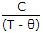for ferro-electric materials.

A. Both A and R are true and R is correct explanation of A
B. Both A and R are true but R is not correct explanation of A
C. A is true but R is false
D. A is false but R is true

Q.34. Hydrogen is used in

A. large size transformers
B. MCB
C. large size generators
D. circuit breakers

Q.35. If a piece of wood is placed in a magnetic field

A. the magnetic field will not be affected
B. the magnetic lines of force will bend towards the piece
C. the magnetic lines of force will bend away from the piece
D. the flux may increase or decrease

Q.36. The number of valence electrons in donor impurity are

A. 5
B. 4
C. 3
D. 1

Q.37. In a true valence crystal, the bonding between the atoms is accomplished by the sharing of valence electrons.

A. True
B. False

Q.38. Which of the following are donor impurities?

1. Gold
2. Phosphorus
3. Boron
4. Antimony
5. Arsenic
6. Induim

Select the correct answer using the following codes

A. 1, 2, 3
B. 1, 2, 4, 6
C. 3, 4, 5, 6
D. 2, 4, 5

Q.39. If n denotes principal quantum number, the angular quantum number l = 0, 1… (n – 1)

A. True
B. False

Q.40. Assertion (A): In a perfect capacitor, the current density is given by ω ∈0E0rcos(ωt + 90°), where ∈r is real part of dielectric constant.

Reason (R): In a perfect capacitor, dielectric losses are zero

A. Both A and R are true and R is correct explanation of A
B. Both A and R are true but R is not correct explanation of A
C. A is true but R is false
D. A is false but R is true

Q.41. Assertion (A): For a solenoid having N turns,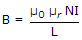.

Reason (R): For all magnetic materials, B increases linearly with I.

A. Both A and R are true and R is correct explanation of A
B. Both A and R are true but R is not correct explanation of A
C. A is true but R is false
D. A is false but R is true

Q.42. Assertion (A): If E is available energy state eV and EF is Fermi level, then probability of energy state E being occupied = 0.5.

Reason (R):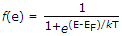A. Both A and R are true and R is correct explanation of A
B. Both A and R are true but R is not correct explanation of A
C. A is true but R is false
D. A is false but R is true

Q.43. Assertion (A): At a temperature of 1000 K, thermionic emission current is about 0.1 A/cm2 of surface.

Reason (R): Thermionic emission current is given by Ith = SA0 T2 e-Ew/kT

A. Both A and R are true and R is correct explanation of A
B. Both A and R are true but R is not correct explanation of A
C. A is true but R is false
D. A is false but R is true

Q.44. At room temperature, the current in intrinsic semiconductor is due to

A. holes
B. electrons
C. ions
D. holes and electrons

Q.45. Ferroelectric materials are those which

A. cannot be polarized
B. have a permanent polarization
C. have ae equal to zero
D. have μp = 0

Q.46. Assertion (A): Power loss in a conductor of resistance P = I2R.

Reason (R): When a conductor is carrying current with current density J as a result of applied field E, then heat developed/m3/ second = JE.

A. Both A and R are true and R is correct explanation of A
B. Both A and R are true but R is not correct explanation of A
C. A is true but R is false
D. A is false but R is true

Q.47. Assertion (A): For Diamond, μr = 1

Reason (R): Diamond is a diamagnetic material.

A. Both A and R are true and R is correct explanation of A
B. Both A and R are true but R is not correct explanation of A
C. A is true but R is false
D. A is false but R is true

Q.48. In crystalline solids, atoms are stacked in a regular manner.

A. True
B. False

Q.49. The temperature below which certain materials are antiferromagnetic and above which they are paramagnetic is called

A. Weiss temperature
B. Curie temperature
C. Neel temperature
D. None of the above

Q.50. In metals, the average drift velocity of electron per unit field is called mobility

A. True
B. False

Q.51. Ferrites have

A. a low copper loss
B. low eddy current loss
C. low resistivity
D. higher specific gravity compared to iron

Q.52. In intrinsic semiconductors, the number of free electrons is equal to the number of mobile holes.

A. True
B. False

Q.53. When a material becomes a superconductor, its resistivity becomes

A. very small
B. zero
C. about 10% of normal value
D. about 20% of normal value

Q.54. In a piezoelectric crystal, the application of mechanical force will cause

A. plastic deformation of crystal
B. magnetic dipoles in the crystal
C. electrical polarization in the crystal
D. shift in Fermi level

Q.55. Consider the following statement
If an electric field is applied to an n type semiconductor bar, the electrons and holes move in opposite directions due to their opposite charges. The net currents is

1. both due to electrons and holes with electrons as majority carriers
2. sum of hole and electron currents
3. difference between electron and hole currents.

Which of above statements are correct?

A. 1 only
B. 1 and 2
C. 2 only
D. 3 only

Q.56. As the temperature of a pure silicon specimen is increased

A. the number of free electrons increases
B. the number of holes decreases
C. the number of holes increases
D. the number of free electrons and holes increases

Q.57. Assertion (A): In many materials, dielectric polarization causes mechanical distortion.

Reason (R): If dielectric polarization causes mechanical distortion then mechanical distortion must cause dielectric polarization.

A. Both A and R are true and R is correct explanation of A
B. Both A and R are true but R is not correct explanation of A
C. A is true but R is false
D. A is false but R is true

Q.58. Semi conducting materials are all

A. elements
B. compounds
C. elements or compounds
D. elements or mixtures

Q.59. Silicon and germanium atoms combine orderly into array of atoms located in repetitive geometric pattern. This pattern is called

A. crystal
B. covalent bond
C. molecule
D. valence bond

Q.60. In a pure sample of silicon

A. the number of holes and free electrons is always equal
B. the number of free electrons is more than the number of holes
C. the number of free electrons and holes is equal at low temperature
D. the number of free electrons is less than number of holes

Q.61. Orbital magnetic dipole moment is due to the motion of electron in its orbit around nucleus.

A. True
B. False

Q.62. Assertion (A): ∈r can be determined experimentally by measuring the capacitance of a parallel plate capacitor with and without the dielectric.

Reason (R): Capacitance of a parallel plate capacitor =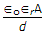.

A. Both A and R are true and R is correct explanation of A
B. Both A and R are true but R is not correct explanation of A
C. A is true but R is false
D. A is false but R is true

Q.63. In a homogeneous magnetic field H of a solenoid, the core carries a magnetic dipole moment M per unit volume equal to

A. μ0 H
B. μr H
C. r – 1) H
D. μoμr H

Q.64. The field pattern of a solenoid is similar to that of bar magnet.

A. True
B. False

Q.65. Assertion (A): In a parallel plate capacitor having area of plate 1 m2 and plate separation 1 m, the capacitance C and resistance R are given by C = ∈0r and R =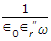where ω is frequency, ∈r and ∈r are real and imaginary parts of dielectric constant.

Reason (R): The imaginary part of electric constant is responsible for dielectric losses.

A. Both A and R are true and R is correct explanation of A
B. Both A and R are true but R is not correct explanation of A
C. A is true but R is false
D. A is false but R is true

Q.66. Assertion (A): Silicon is the most favoured semiconductor material.

Reason (R): PIV for silicon diode is more than that for germanium diode.

A. Both A and R are true and R is correct explanation of A
B. Both A and R are true but R is not correct explanation of A
C. A is true but R is false
D. A is false but R is true

Q.67. Assertion (A): When cathode temperature is increased from 2500 K to 2550 K, thermionic emission current may increase by about 50%.

Reason (R): Thermionic emission current ∝ T2 e-Ew/kT.

A. Both A and R are true and R is correct explanation of A
B. Both A and R are true but R is not correct explanation of A
C. A is true but R is false
D. A is false but R is true

Q.68. In molecules of ionic crystals, orientation polarization is zero.

A. True
B. False

Q.69. Assertion (A): CO2 has no resultant dipole moment but CO has a dipole moment.

Reason (R): The structure of CO2 is O = C = O.

A. Both A and R are true and R is correct explanation of A
B. Both A and R are true but R is not correct explanation of A
C. A is true but R is false
D. A is false but R is true

Q.70. For a photoconductor with equal electron and hole mobilities and perfect ohmic contacts at the ends, an increase in intensity of optical illumination results in

A. a change in o.c voltage
B. a change in s.c current
C. decrease in resistance
D. increase in resistance

Q.71.  A permeable substance is one

A. which is strong magnet
B. which is a weak magnet
C. which is a good conductor
D. through which magnetic lines of force can pass easily

Q.72. When subjected to alternating stresses, an insulating material is characterised by complex dielectric constant ∈r – jr . In such materials, the dielectric losses under alternating stress is proportional

A. r
B. both ∈r and ∈r
C. r
D. (∈r)2

Q.73. The classes of solid dielectrics are

A. A, B, C, D, E, F, H
B. A E B F H C D
C. Y A E B F H C
D. A E B F H C

Q.74 A 200 turn coil has a self inductance of 10 mH. If the number of turns is doubled, all other quantities remaining the same, the self inductance will be

A. 2.5 mH
B. 5 mH
C. 20 mH
D. 40 mH

Q.75. A material which is lightly repelled by a magnetic field is known as

A. diamagnetic material
B. non-magnetic material
C. ferromagnetic material
D. paramagnetic material

Q.76. Which of the following is the poorest conductor of electricity?

A. Aluminium
B. Nichrome
C. Copper
D. All of the above

Q.77. The magnetic moment in units of Bohr magneton of a ferrous ion in any ferrite is

A. zero
B. 2
C. 4
D. 6

Q.78. The relationship of hysteresis loss to maximum flux density was first determined by

A. C. Steinmetz
B. G.R. Kirchoff
C. Mr. Laplace
D. Mr. Ampere

Q.79. In metals the thermal conductivity K and electrical conductivity σ are related as (K/σT) = L, L is known as

A. Lattice constant
B. Lorentz number
C. Lanevin function
D. Larmor number

Q.80. Assertion (A): If WF is Fermi energy, v is velocity of electron and m is mass of electron, then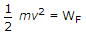.

Reason (R): Conductivity of a metal depends on temperature and impurity concentration.

A. Both A and R are true and R is correct explanation of A
B. Both A and R are true but R is not correct explanation of A
C. A is true but R is false
D. A is false but R is true

Q.81. Dielectric constant of transformer oil is about

A. 1
B. 4
C. 10
D. 20

Q.82. Assertion (A): When an electron is revolving in its orbit and magnetic field is also present, the angular frequency of electron will be affected by the magnetic field.

Reason (R): In the case of electron revolving in its orbit in the presence of magnetic field, the orbital magnetic dipole moment is not affected by the presence of magnetic fie

A. Both A and R are true and R is correct explanation of A
B. Both A and R are true but R is not correct explanation of A
C. A is true but R is false
D. A is false but R is true

Q.83. In carbon resistor colour code, the third band is red. It indicates

A. two zeros
B. three zeros
C. multiplier is 0.01
D. multiplier is 0.1

Q.84. Rochelle salt is a ferroelectric material.

A. True
B. False

Q.85.  Paramagnetic materials have permanent magnetic dipoles but interaction between neighbouring dipoles is negligible

A. True
B. False

Q.86. Assertion (A): If difference between static and optical dielectric constant is high, the mechanical compressiblity of that material is also high.

Reason (R): For soft ions, ionic polarization per unit field is higher than that of hard ions.

A. Both A and R are true and R is correct explanation of A
B. Both A and R are true but R is not correct explanation of A
C. A is true but R is false
D. A is false but R is true

Q.87. Assertion (A): The optical dielectric constant (i.e., εr at optical frequencies)of ionic dielectrics is less than static dielectric constant.

Reason (R): At optical frequencies, Pi = 0.

A. Both A and R are true and R is correct explanation of A
B. Both A and R are true but R is not correct explanation of A
C. A is true but R is false
D. A is false but R is true

Q.88. A piece of iron is placed in a magnetic field

A. the magnetic field will not be affected
B. the magnetic lines of force will bend to pass through the piece
C. the magnetic lines of force will bend away from the piece
D. any of the above depending on type of iron

Q.89. Assertion (A): Magnetism can be explained by the theory of magnetic domains.

Reason (R): The existence of magnetic domains is evident from the Bitter powder patterns

A. Both A and R are true and R is correct explanation of A
B. Both A and R are true but R is not correct explanation of A
C. A is true but R is false
D. A is false but R is true

Q.90. Ferromagnetic materials

A. shows  spontaneous magnetisation below a certain temperature
B. have resistivity very high as compared to that of iron
C. can be used even at microwave frequencies
D. all of the above

Q.91. In a specimen of ferromagnetic material, as the flux density is increased from zero to 2.5 T, µr will

A. increase
B. decrease
C. first decrease then increase
D. first increase then decrease

Q.92. Assertion (A): Relaxation time and mean time between collisions are equal when scattering is isotropic.

Reason (R): Mean free path of an electron λ, velocity of electron v and mean time between collisions τc are related as λ = vTc.

A. Both A and R are true and R is correct explanation of A
B. Both A and R are true but R is not correct explanation of A
C. A is true but R is false
D. A is false but R is true

Q.93. Assertion (A): Copper is a monoatomic solid.

Reason (R): In a metal the valence electrons belong to all atoms.

A. Both A and R are true and R is correct explanation of A
B. Both A and R are true but R is not correct explanation of A
C. A is true but R is false
D. A is false but R is true

Q.94. The heat developed per m3 per second (W) in a conductor having a conductivity σ and a field E is

A. W = σE2
B. W = σE
C. W =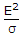D. W = (σE)0.5